# 如何用 Python 执行常见的 Excel 和 SQL 任务

### 导入数据

（不用担心，如果你想跳过这个部分，可以的！原始的 csv 文件在这里，你可以随意下载，如果你宁愿开始这个练习而不从网络上获取数据，或者你可以 git clone 整个存储库](https://github.com/Rogerh91/codelove-tutorials/tree/master/Excel_SQL_to_Python)。）

```import pandas as pd
import numpy as np
import requests
from bs4 import BeautifulSoup
import re```

```r = requests.get('https://en.wikipedia.org/wiki/List_of_countries_by_GDP_(nominal)_per_capita')

gdptable = r.text
soup = BeautifulSoup(gdptable, 'lxml')
table = soup.find('table', attrs = {"class" :"wikitable sortable"})

for tx in table.findAll('th'):

data =[]
for rows in table.findAll('tr'):
row={}
i=0
for cell in rows.findAll('td'):
i+=1
if len(row)!=0:
data.append(row)
print(data)```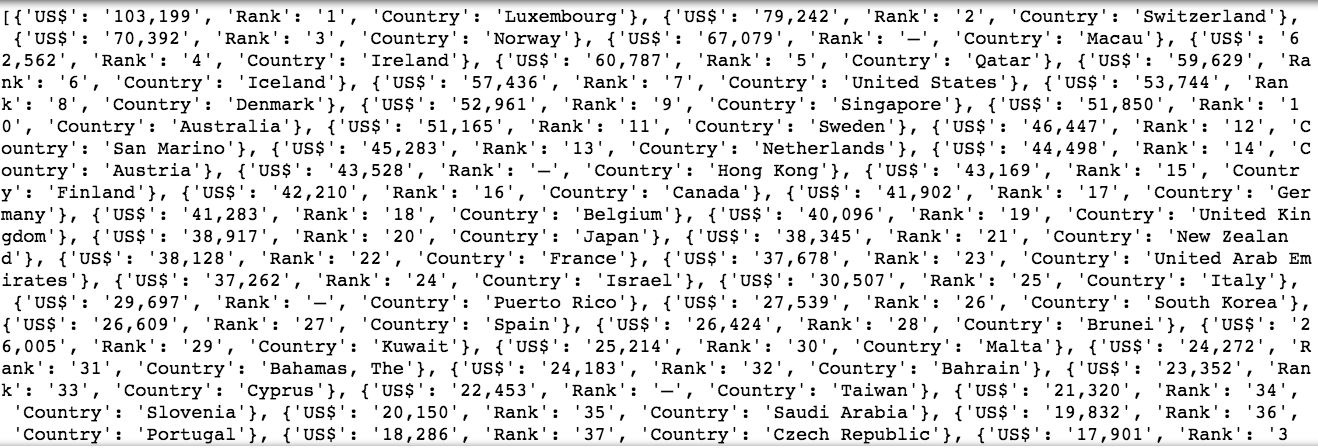`gdp = pd.DataFrame(data)`

### 快速查看数据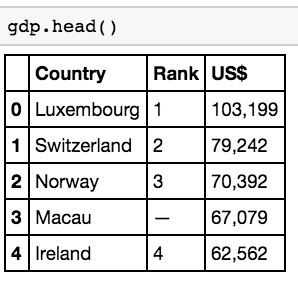### 重命名列

```gdp = gdp.rename(columns = {'US
```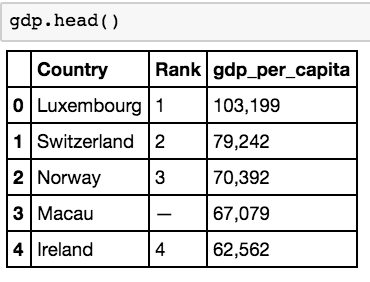### 删除列

`del gdp['Rank']`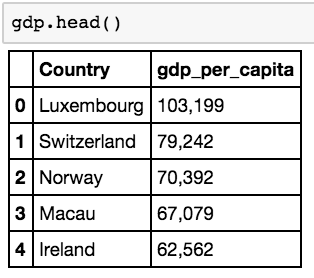### 在列中转换数据类型

`gdp['gdp_per_capita'] = gdp['gdp_per_capita'].apply(lambda x: re.sub(',','',x))`

re.sub 方法本质上是使用空格替换逗号。以下教程详细介绍了 re库 的各个方法。

`gdp['gdp_per_capita'] = gdp['gdp_per_capita'].apply(pd.to_numeric)`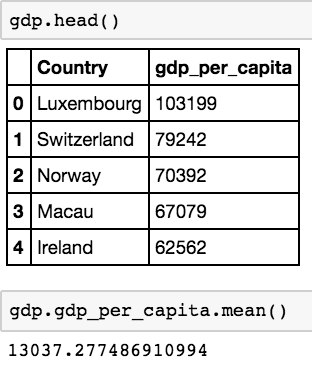### 选择/过滤数据

`gdp50000 = gdp[gdp['gdp_per_capita'] > 50000]`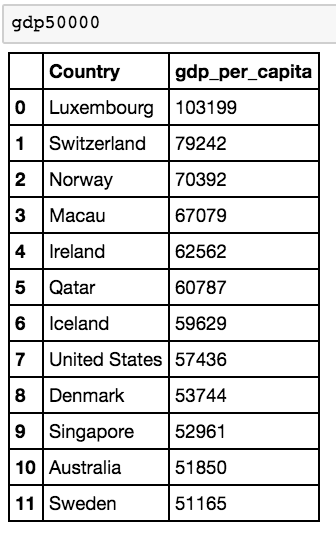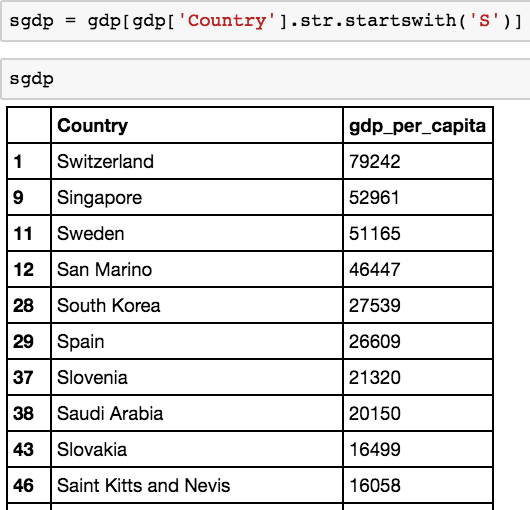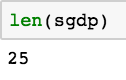`sand500gdp = gdp[(gdp.gdp_per_capita > 50000) & (gdp.Country.str.startswith('S'))]`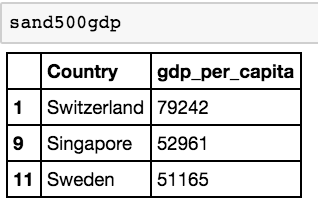`sor500gdp = gdp[(gdp.gdp_per_capita > 50000) | (gdp.Country.str.startswith('S'))]`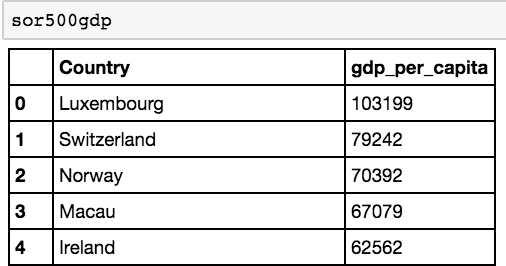### 用计算机来处理数据

`gdp50000.gdp_per_capita.sum()`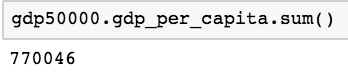### 数据可视化（图表/图形）

`gdp50000.hist()`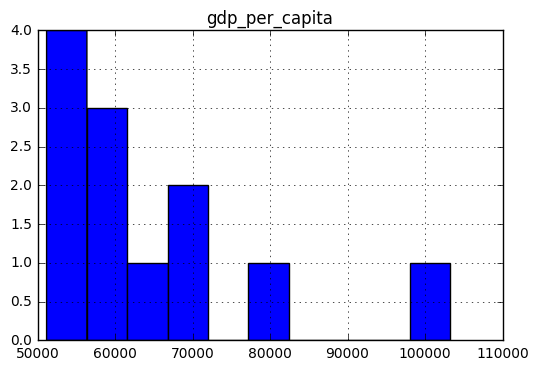### 分组和连接数据

Pandas 和 Python 共享了许多从 SQL 和 Excel 被移植的相同方法。可以在数据集中对数据进行分组，并将不同的数据集连接在一起。你可以看看这里的文档。你会发现，由 Pandas 中的merge 方法提供的连接功能与 SQL 通过 join 命令提供的连接功能非常相似，而 Pandas 还为过去在 Excel 中使用数据透视表的人提供了 pivot table 方法

`country = pd.read_csv("Country.csv")`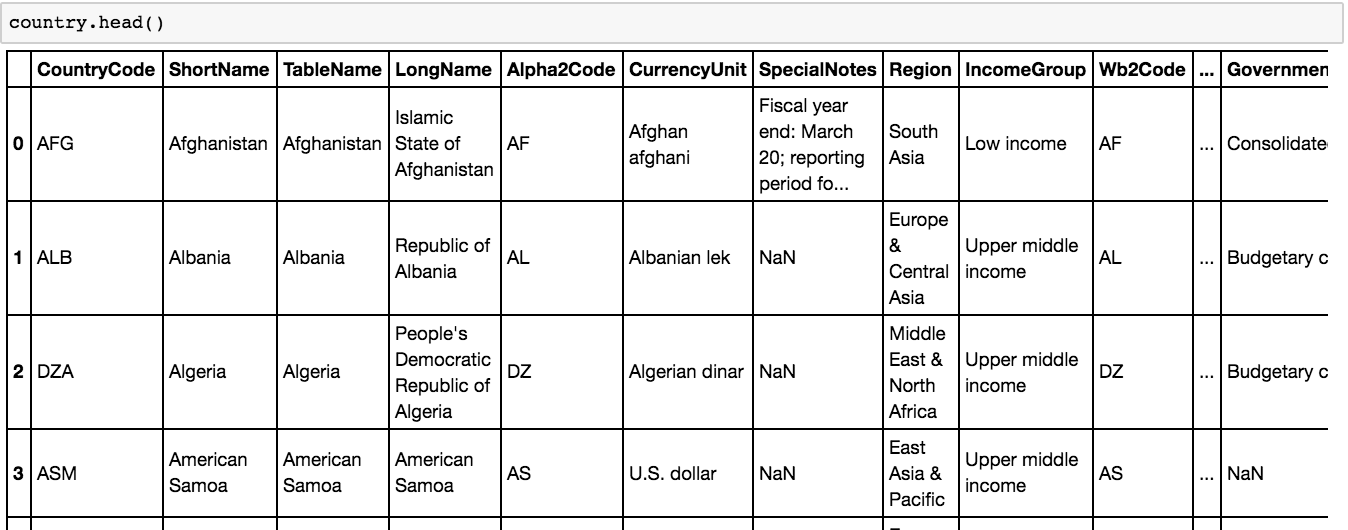`gdpfinal = pd.merge(gdp,country, how = 'inner', left_on='Country', right_on = 'TableName')`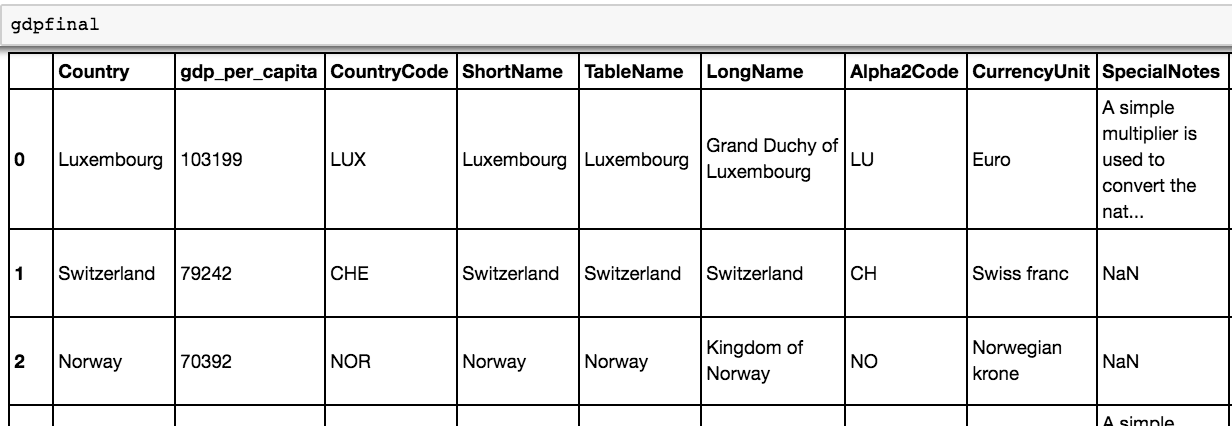`gdpregion = gdpfinal.groupby(['Region']).mean()`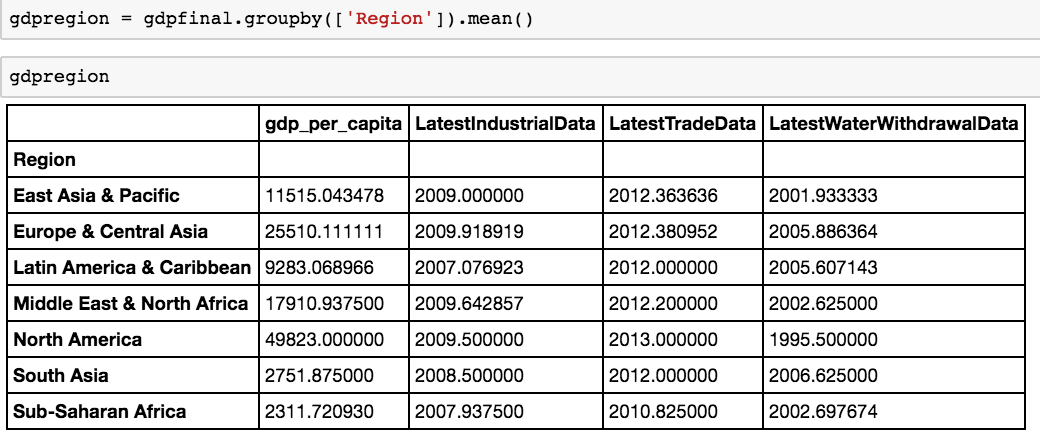```gdppivot = gdpfinal.pivot_table(index=['Region'], margins=True, aggfunc=np.mean)

gdppivot```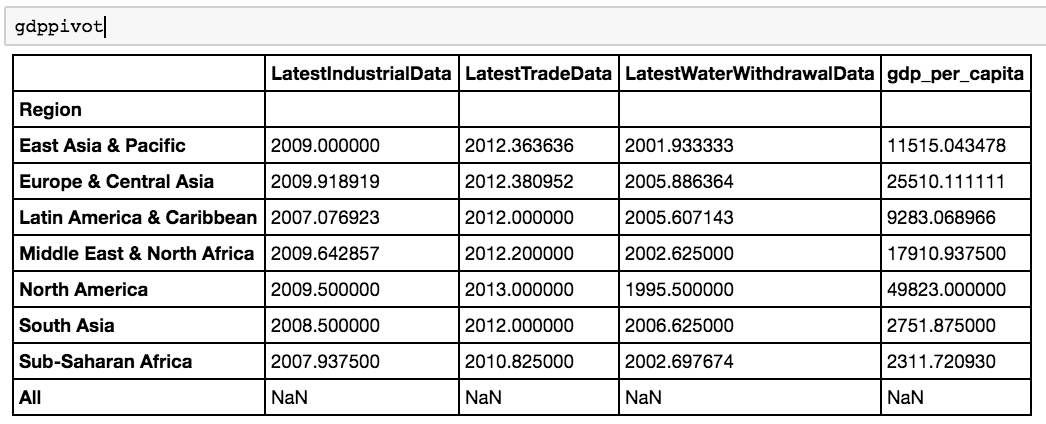```gdppivot.drop(['LatestIndustrialData', 'LatestTradeData', 'LatestWaterWithdrawalData'], axis=1, inplace=True)

gdppivot```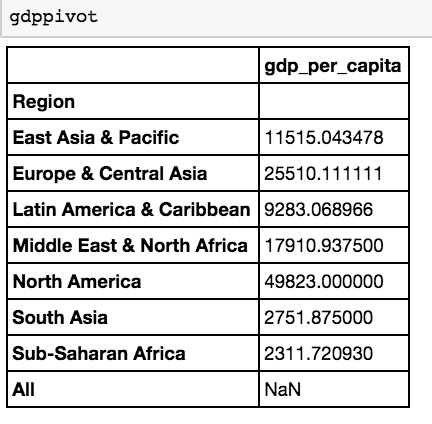:’gdp_per_capita’})### 删除列### 在列中转换数据类型

re.sub 方法本质上是使用空格替换逗号。以下教程详细介绍了 re库 的各个方法。### 选择/过滤数据### 用计算机来处理数据### 数据可视化（图表/图形）### 分组和连接数据

Pandas 和 Python 共享了许多从 SQL 和 Excel 被移植的相同方法。可以在数据集中对数据进行分组，并将不同的数据集连接在一起。你可以看看这里的文档。你会发现，由 Pandas 中的merge 方法提供的连接功能与 SQL 通过 join 命令提供的连接功能非常相似，而 Pandas 还为过去在 Excel 中使用数据透视表的人提供了 pivot table 方法Python

## Superset在windows下的安装配置

2018-3-24 23:25:29

Python

## Python数据化运营分析案例：销售预测

2018-3-27 2:00:27

0 条回复 A文章作者 M管理员
暂无讨论，说说你的看法吧